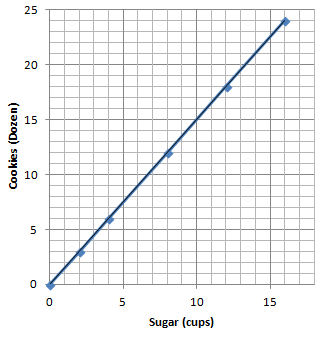# Proportional Relationships

## Objective

Represent proportional relationships in graphs.

## Common Core Standards

### Core Standards

?

• 7.RP.A.2 — Recognize and represent proportional relationships between quantities.

• 7.RP.A.2.A — Decide whether two quantities are in a proportional relationship, e.g., by testing for equivalent ratios in a table or graphing on a coordinate plane and observing whether the graph is a straight line through the origin.

• 7.RP.A.2.D — Explain what a point (x, y) on the graph of a proportional relationship means in terms of the situation, with special attention to the points (0, 0) and (1, r) where r is the unit rate.

?

• 6.RP.A.3.A

## Criteria for Success

?

1. Graph a proportional relationship in a coordinate plane using a table of values.
2. Identify key features of the graph of a proportional relationship: a straight line that passes through the origin.
3. Understand the graph of a proportional relationship to represent the set of equivalent ratios of the two quantities, represented as coordinate points.
4. Interpret the coordinate point $(x,y)$ as it relates to the context of the situation.

## Tips for Teachers

?

• Lessons 6 and 7 focus on representing proportional relationships in the coordinate plane. Students may need to review concepts and skills around the coordinate plane in order to fully access this lesson.
• The following materials are used or would be useful in this lesson: graph paper, rulers.

#### Fishtank Plus

• Problem Set
• Student Handout Editor
• Vocabulary Package

## Anchor Problems

?

### Problem 1

An amusement park sells tickets that can be used to go on rides and play games. The cost of the tickets is proportional to the number of tickets purchased. The table below shows how many tickets can be purchased for different amounts of money.

 # of Tickets Cost (\$) 2 3 4 6 6 9 8 12 10 15

Graph the relationship in a coordinate plane. What features do you notice about the graph of a proportional relationship?

### Problem 2

Below is a graph modeling the amount of sugar required to make Grandma’s special chocolate chip cookies.1. Record the coordinate points from the graph. What do these ordered pairs represent?
2. Grandma has 1 remaining cup of sugar. How many dozen cookies will she be able to make? Plot the point on the graph above.
3. How many dozen cookies can Grandma make if she has no sugar? Can you graph this on the line in the coordinate plane above? What do we call this point?

#### References

EngageNY Mathematics Grade 7 Mathematics > Module 1 > Topic B > Lesson 10Example 2

Grade 7 Mathematics > Module 1 > Topic B > Lesson 10 of the New York State Common Core Mathematics Curriculum from EngageNY and Great Minds. © 2015 Great Minds. Licensed by EngageNY of the New York State Education Department under the CC BY-NC-SA 3.0 US license. Accessed Dec. 2, 2016, 5:15 p.m..

## Problem Set

?The following resources include problems and activities aligned to the objective of the lesson that can be used to create your own problem set.

• Include review problems of prior skills such as graphing coordinate points in the coordinate plane, determining and setting up appropriate scale intervals, naming coordinate points in the coordinate plane, distinguishing between points on the $x$-axis and those on the $y$-axis, etc.
• Include problems where students are given a table of values and are asked to represent the relationship in a graph.
• Include problems where students are given a graph of a proportional relationship and must write a table of values to accompany it.
• Include problems where students interpret coordinate points $(x,y)$ from both graphs and tables

?

The graph below shows the amount of time a person can shower with a given amount of water.1. Can you determine by looking at the graph whether the length of the shower is proportional to the number of gallons of water? Explain how you know.
2. How long can a person shower with 15 gallons of water?
3. How many gallons of water are used during a 15 minute shower?
4. What are the coordinates of point A? Describe point A in context of the problem.

#### References

EngageNY Mathematics Grade 7 Mathematics > Module 1 > Topic B > Lesson 10Exercise 1

Grade 7 Mathematics > Module 1 > Topic B > Lesson 10 of the New York State Common Core Mathematics Curriculum from EngageNY and Great Minds. © 2015 Great Minds. Licensed by EngageNY of the New York State Education Department under the CC BY-NC-SA 3.0 US license. Accessed Dec. 2, 2016, 5:15 p.m..

?